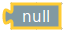en
uk

# LOGIC

Logic blocks are generally used to control conditional blocks and repeat blocks.

Here's an example: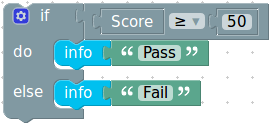If the score is more than or equal to 50, the condition is true, and the text "Pass" is printed. If the score is not greater than or equal to 50, the condition is false, and "Fail" is printed.

Boolean values can also be stored in variables and passed to functions, the same as numbers, text, and list values. Leaving an input empty is not recommended.

Values

A single block, with a drop-down specifying either true or false, can be used to get a Boolean value: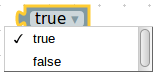Comparisons

There are six comparison operators. Each takes two inputs and returns true or false depending on how the inputs compare with each other.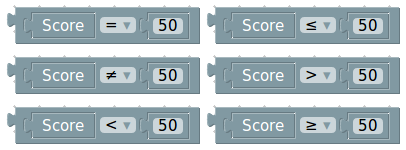The six operators are: equals, not equals, less than, greater than, less than or equal, greater than or equal.

Logical operations

The and block will return true only if both of its two inputs are also true.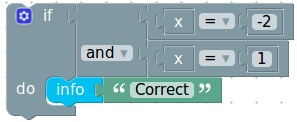The or block will return true only if either of its two inputs are true.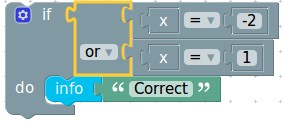Not

The not block converts its Boolean input into its opposite. For example, the result of: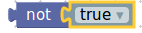is false.

Ternary operator

The ternary block acts like a miniature if-else block. It takes three inputs; the first input is the Boolean condition to test, the second input is the value to return if the test was true, the third input is the value to return if the test was false. For example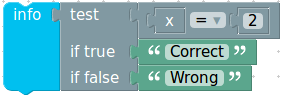Null

The null block allows the value to be set as Null instead of the usual possible values of the data type.